×

Search anything:

# Array in Python

#### Python Software EngineeringGet this book -> Problems on Array: For Interviews and Competitive Programming

In this article, one can understand the most awaiting information of arrays in Python, their usage, types, advantages, and many others.

TABLE CONTENTS
Introduction
Why use Arrays in Python?
What is an Array in Python?
Is Python list the same as an Array?
Creating an array in Python
Accessing an element in array
Functions in array module
Types of Arrays in Python
Conclusion

# INTRODUCTION

This article will give a clear view of the Arrays in Python, but before getting started let us visualize an example that will help one to understand the use of Arrays in Python. For an instance let us imagine that we have been told to store integers from 1 to 1000.

What we will do? Will we be able to remember all the variable names?
Of Course not!!... Then what can be done??
We will not able to remember the name of the 1000 variable explicitly, So in that case we can easily save them using the array.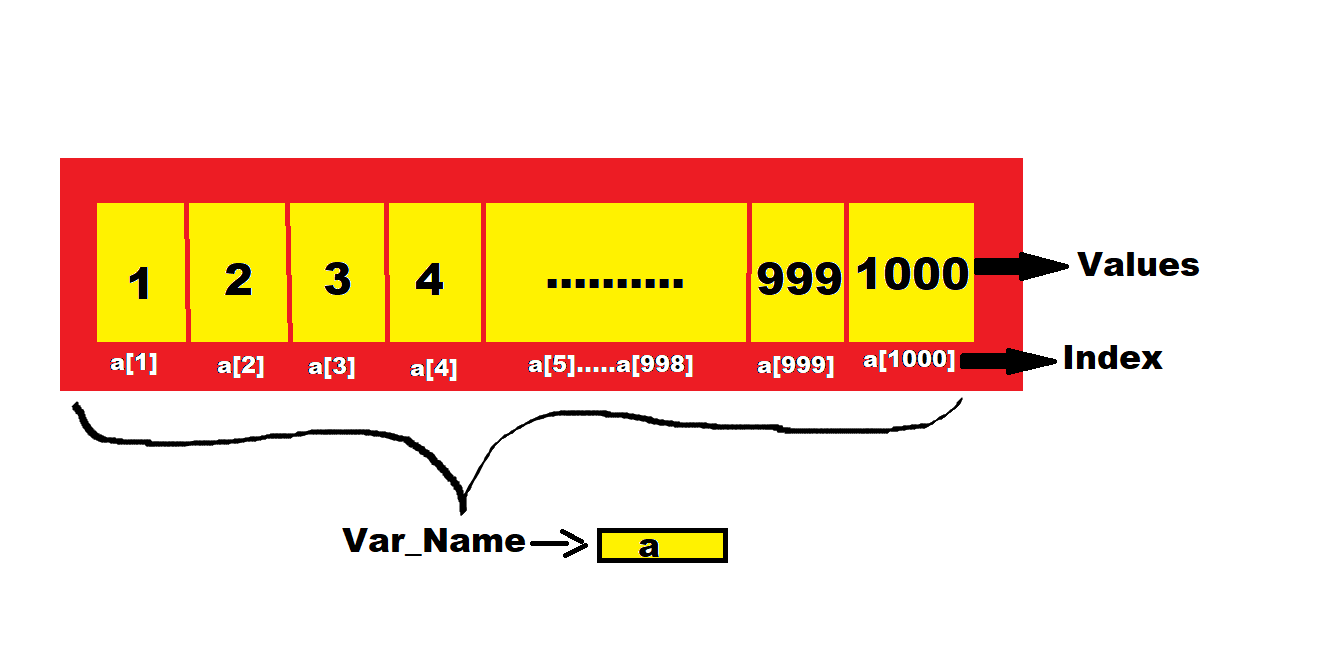So let us get started with why to use Arrays in Python now:

### Why to use Arrays in Python

Arrays help us to reduce the size of the code and as we all know python has the most simple syntax compared to the other programming languages which will help us to get rid of other problematic syntaxes. So in short Arrays in Python saves a lot of time. Now we have got an idea about the importance of Array in Python, let us study in detail about what is Array in Python.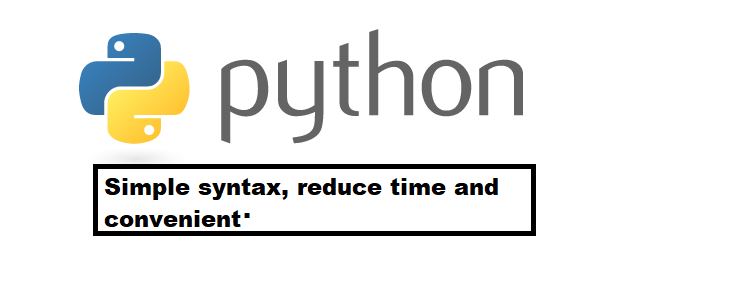# What is an Array in Python?

Python arrays are a data structure like lists. They contain several objects that can be of different data types. In addition, Python arrays can be iterated and have several built-in functions to handle them. Python has several built-in data structures, such as arrays. Arrays give us a way to store and organize data, and we can use the built-in Python methods to retrieve or change that data. For example, if you have a list of student names that you want to store, you may want to store them in an array.

Arrays are useful if you want to work with many values of the same Python data type. This might be student names, employee numbers, etc. They allow you to store related pieces of data that belong. And they make it easy to perform the same operation on multiple values at the same time.

# Is Python list the same as an Array?

Python Arrays and lists similarly are store values. But there is a key difference between the two is in the values that they store. A list can store any type of values such as integers, strings, etc. Arrays, on the other hand, stores single data type values. Therefore, you can have an array of integers, an array of strings, etc.

Python also provides Numpy Arrays which are a grid of values used in Data Science. In simple List stores different data types but arrays can store only same data types.

# Creating an array in Python

Arrays in Python can be created after importing the array module as follows:

1. Import array as arr

The array(data type, value list) function takes two parameters, the first being the data type of the value to be stored and the second being the value list. The data type can be anything such as int, float, double, etc. Please make a note that arr is the alias name and is for ease of use. You can import without an alias as well. There is another way to import the array module which is:

1. From array import *

This means you want to import all functions from the array module.
The following syntax is used to create an array.

### Syntax:

``````a=arr.array(data type,value list)           #(when you import using arr alias)
``````

OR

``````a=array(data type,value list)              #(when you import using array import* )
``````

let us see the implementation to illustration:

``````# Program to create a array
# import array module
import array as arr
# d is the datatype for float
values_float = arr.array('d', [3.7, 6.6, 7.8, 8.9])
# u is the datatype for
char values_char = arr.array('u', ['a', 'b', 'c', 'd'])
# printing float array using for loop
print("ARRAY 1 : ", end="  ")
for i in range(0, 4):
print(values_float[i], end="   ")
print()
# printing character array using for loop
print("ARRAY 2 : ", end="   ")
for i in range(4):
print(values_char[i], end="   ")
print()
``````

### OUTPUT

``````ARRAY 1: 3.7  6.6  7.8  8.9
ARRAY 2:  a    b    c    d
``````

# Accessing an element in the array

Let us now have a look at how arrays help in viewing a shop's, Apple's stock price for 5 days using arrays in python: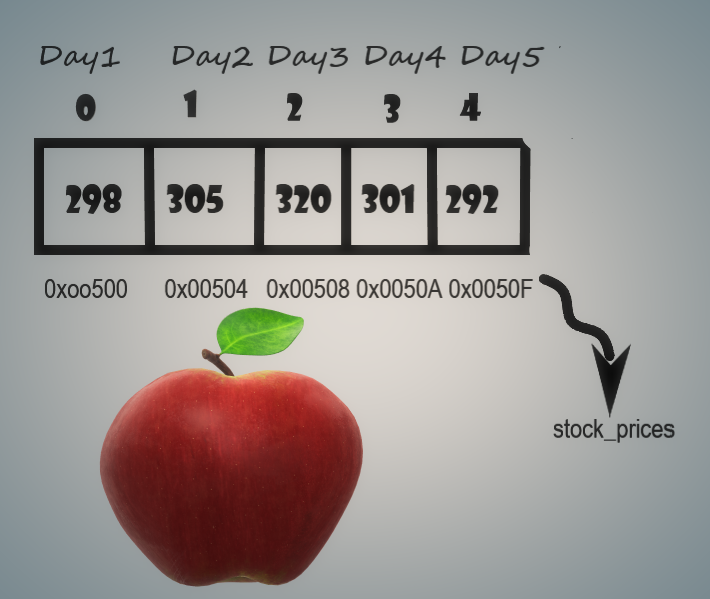``````stock_prices=[298,305,320,301,292]
``````

The above Representation of the Array elements depicts some facts about arrays in python, which are significant for mastering them.

Indexing on Arrays begins from 0. To access the elements, index plays a vital role. For example, if you want to retrieve the price of
day 1 we will use stock_prices which will lead you to the day one price which is 298. Similarly on day 3, we will use stock_prices.

When you look at the internal memory presentation it will look something like the above-depicted picture, when I say memory I'm referring to a RAM, When we are running a code the code is run by the CPU on our computer but it is using a RAM to store all the variables and data, stock_prices in my python program is a variable which it will store on these types of memory location which have addresses(eg:0x00500)so this hexadecimal number is the addresses on RAM, now if we can open our computer and if we look at the RAM and let's say there is some way of visualizing what is stored on that RAM, then we can notice that the numbers are stored in bits of ones and zeros the representation which I depicted above is a simple representation but in reality, we convert that number into a binary number:

``````298 = 100101010
``````

There is some formula one has to apply to convert a number into a binary number, here every single digit is a bit which could be either 0 or 1. When we have integer number we use four bytes to store the number. Hence we store 298 as below:

``````298=00000000 00000000 00000001 00101010
``````

1 byte is 8 bits, so we can see that 298 has 4 bytes. These 4 bytes are stored on the memory location, so 298 is stored like the above quoted.

So in reality the stock prices of apple are stored in the way as depicted below: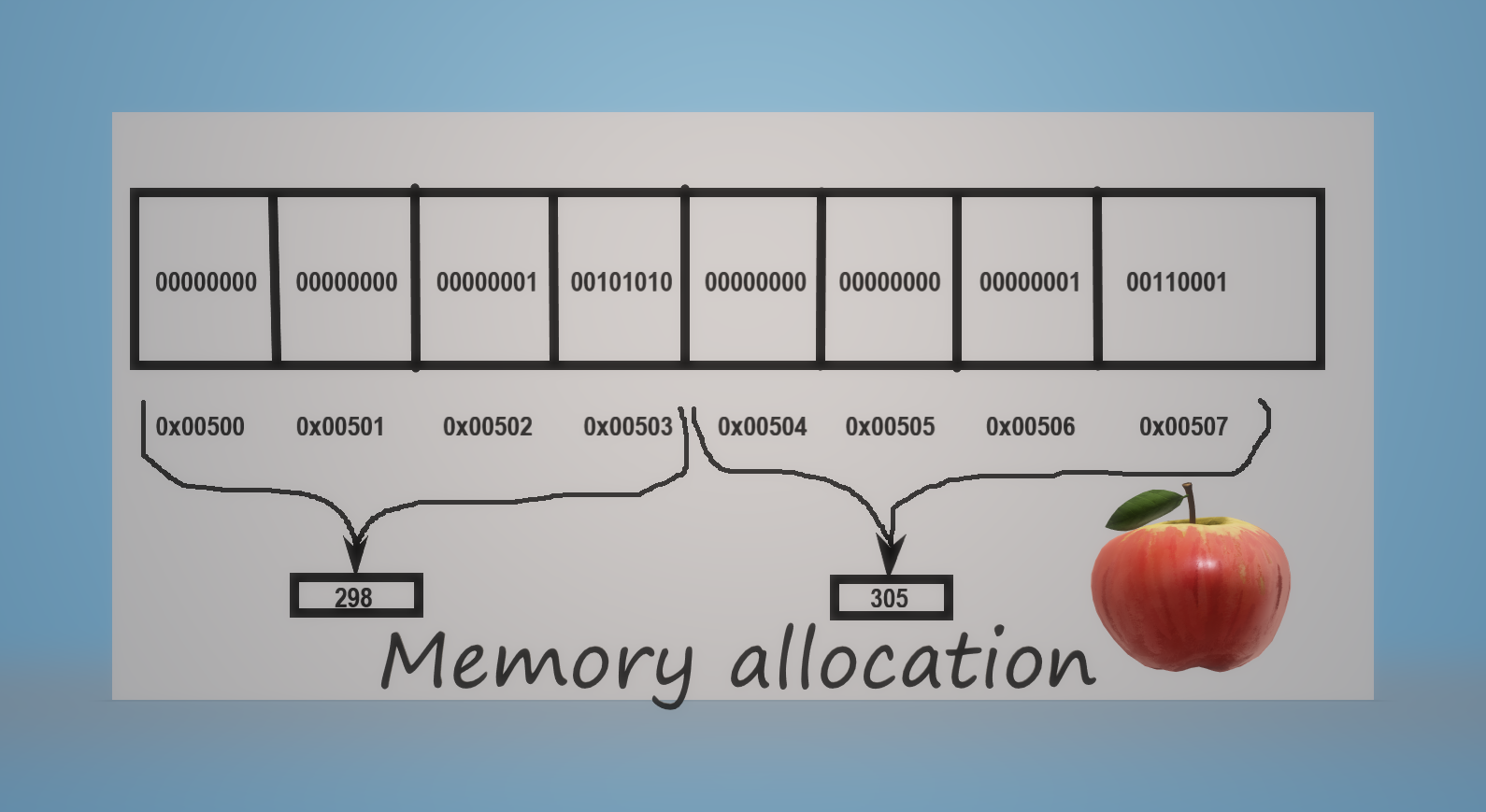Now we can see some Scenario's for our understanding:

1. What was the price on day 3?
In this case, we can easily find using the index of the arrays, we know that the index values in arrays start with 0, and hence to find the stock_prices of day 3 we have to use the index 2 that will give us
320 (price on day 3).

Now let us see how it works:

``````Adress (nth)= Base address + (n x Size of the data type)
``````

where n is the desired element's index, Base Address is the 0x00500, Size=4(since the array is of integer type, the size is 4)

NOW LET US ACCESS THE THIRD ELEMENT:
n=3;

``````Address(3rd) = 0x00500 + (3 x (4))
= 0x00500 + 12
=  0x0050A
``````

Now, we can easily access the element present at that address. The time complexity will be 0(1) as it takes a constant time.

1. SCENARIO two: On what day price was 301?
A simple python program can be running a for loop on your values and comparing these values one by one to check when we get 301 we will return that value and print it,(which will print the day 3)
``````for i in range(len(stock_prices)):
if stock_prices[i]=301:
return i
``````
1. SCENARIO THREE: Print all the prices;
In this case, we can use a for loop like the following:
``````for price in stock_prices:
print(price)
``````

From the above scenario's one can get to know how to access the elements in the array. Now let us see some functions which are in the array module:

# Functions in the array module

### 1.To find the length of the array:

syntax:

``````len(array_name)
``````

The len function is used to find the length of the array.

### 2.To reverse the array:

syntax:

``````array name.reverse()
``````

The reverse function is used to reverse the elements in the array.

### 3.Adding/ Changing elements of an Array:

syntax:

``````array_name.append()
array_name.extend()
``````

We can add value to an array by using the append(), extend() and the insert (i,x) functions.
The append() function is used when we need to add a single element at the end of the array.

### Below is the implementation:

``````#Imported the array module
import array as arr
#Created a float array
values_float = arr.array('d',[3.3,3.5,6.5,7.8])
#To find the length of the array
print("The length of the array is: ",end=" ")
print(len(values_float))
#Print the Original array
print("The Original array: ",end=" ")
print(values_float, end=" ")
print()
#function to reverse the array elements
values_float.reverse()
print("The Reversed array : ", end=" ")
for i in the range(len(values_float)):
print(values_float[i],end=" ")
print()
#To add an element in the array
values_float.append(8.1)
print("The new array : ",end=" ")
print(values_float)
print()
``````

### OUTPUT

``````The length of the array is: 4
The Original array: 3.3 3.5 6.5 7.8
The Reversed array: 7.8 6.5 3.5 3.3
The new array : 3.3 3.5 6.5 7.8 9.1
``````

The above mentioned are just a glimpse of the array module and there are many more functions.

# TYPES OF ARRAYS IN PYTHON

Till now we have only discussed the single-dimensional array, which is the one type of array in python. The second type is a Multi-dimensional array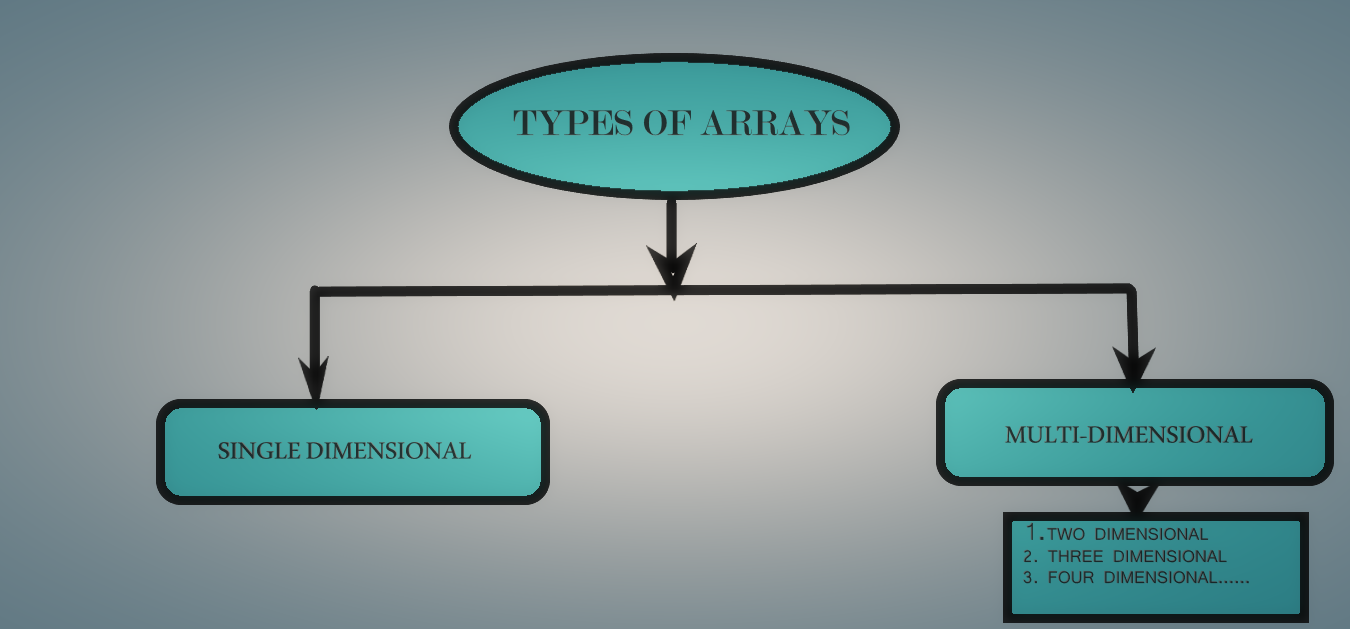Multidimensional arrays in python are the arrays present within the arrays. Multidimensional arrays can be further divided into various types:

• TWO-DIMENSIONAL ARRAY
• THREE-DIMENSIONAL ARRAY
• FOUR DIMENSIONAL ARRAY and the list goes on...

A two-dimensional array has two subscripts[][], the 1st of which indicates the number of rows and the second indicates the number of columns.
A two-dimensional array is commonly referred to as a matrix.

### For example:

``````int num ----> Declaration of the 2-D array of the type int.
``````

Number of Elements =20 x 35 =700 elements can be stored in the num array.

REMARK:

The array module in python doesn't support multidimensional arrays. We need to import another module named Numpy.

Numpy, which stands for Numerical Python, is a library consisting of multidimensional array objects and a collection of routines for processing those arrays. Using NumPy, mathematical and logical operations on arrays can be performed.

# CONCLUSION

Arrays are very flexible data structures in Python that we can use to work with lists of data. In this article at OpenGenus, I have demonstrated how arrays can be used to store multiple values and how they are accessed. I have discussed how to retrieve a Python array using its index numbers. We also covered how to modify items in an array.

I discussed how to add and remove items from an array using the append() and extend() functions, respectively. Now, one can be prepared for a variety of situations where you may need to use an array in Python!

Happy learning everyone!!!!!.....
Let's learn and explore together!!!!....
Have a GOOD DAY!!<3......#### DEEPIKA N

PURSUING MASTERS IN MATHEMATICS AT INDIAN INSTITUTE OF TECHNOLOGY ,ROPAR, PUNJAB(IIT ROPAR).